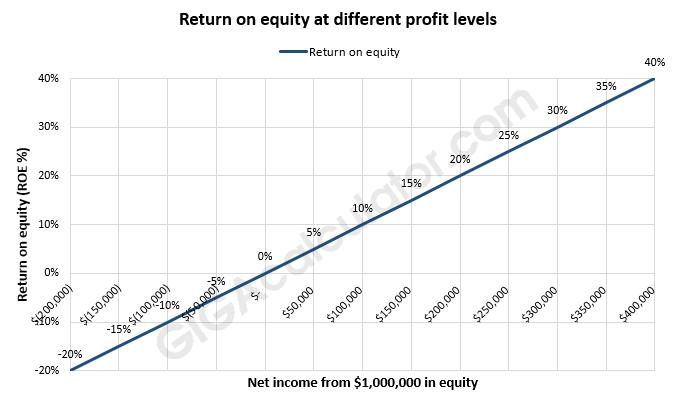# Return on Equity Calculator

Use this ROE calculator to easily calculate ROE (return on equity) based on the net income generated and the total value of the equity of the company or project.

\$
\$
Share calculator:

Embed this tool:
get code

## ROE Formula

The formula for ROE used in our return on equity calculator is simple:

ROE = Net Income / Total Equity

Net income is also called "profit". Both input values are in the relevant currency while the result is a ratio. To get a percentage result simply multiply the ratio by 100. Note that in case of excessive debt the equity might be a negative number, leading to negative ROE.

## How to calculate return on equity?

Return on Equity (ROE) is a metric used to estimate the financial performance of a company in terms of how well a it uses its net assets (equity equals the company's assets minus its debt/liabilities). It is calculated as the company net income (profit) relative to the net value of its assets, or equity. Note the difference between that calculation and the one used to calculate Return on Capital Employed (ROCE) in which liabilities are also considered.

Substituting the relevant values in the above formula is easy. For example, if the net income of a company for the fiscal year is \$100,000 and it used net assets worth \$1,000,000 to produce it, then its return on equity is 1/10 or 10%. You can verify this using our ROE calculator. This result means that the business returns 1 dollar of value for every 10 dollars of net capital assets. As the net equity can fluctuate over the year you can input its arithmetic average instead.The return on equity is also used in calculating the expected growth of a company by multiplying it by the retention ratio, or the percentage of net income that is reinvested by the company to fund future growth. Such calculations are known as "sustainable growth model" analyses.

If you are the sole investor in the company and the company has no outstanding debt or liabilities, then the return on equity is equal to your return on investment (ROI) so you can use this return on equity calculator or a ROI calculator with to the same result.

## What is a good return on equity?

In most mature businesses with a low level of risk a 12-15% annual return on equity is considered pretty decent. Highly-volatile and risky investments, on the other hand, would be expected to bring in 20%, 30% or even higher return to justify the risk-adjusted cost. In this sense "good" is a subjective term that depends on the particular niche, competition, perceived risk, and investor time preference, among others.

For the above reasons ROE is a good measure for comparing companies only when the operate under relatively similar conditions: same industry, same geographical location, same legal and regulatory framework, etc. The more diverse these are, the more you need to consider other metrics on top of calculating ROA. Still, a common "shortcut" is to compare a company's performance to the long-term average ROE of the S&P 500 (which stands at 14%) as acceptable and everything below 10% as quite poor.

#### Cite this calculator & page

If you'd like to cite this online calculator resource and information as provided on the page, you can use the following citation:
Georgiev G.Z., "Return on Equity Calculator", [online] Available at: https://www.gigacalculator.com/calculators/return-on-equity-calculator.php URL [Accessed Date: 09 Jun, 2023].